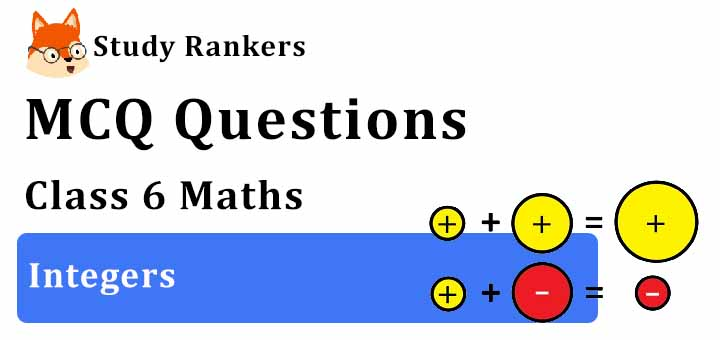## MCQ Questions for Class 6 Maths: Ch 6 Integers1. Product of -140 and +8 is
(a) 1120
(b) 3200
(c) −1120
(d) −3200
► (c) −1120

2. Which of the following is the greatest negative integer?
(a) -100
(b) Does not exist.
(c) -1
(d) -9
► (c) -1

3. Compare pairs of numbers using > or <: 0 _____ -15
(a) <
(b) =
(c) >
(d) None of these
► (c) >

4. (– 4) + (+ 3) = _____
(a) 7
(b) -1
(c) 6
(d) None of these
► (b) -1

5. What is the integer representation of walking 3km south?
(a) Walking 3 km towards the north
(b) -3 km
(c) +3 km
(d) +3
► (b) -3 km

6. Sum of – 30 and – 12 is
(a) -42
(b) 42
(c) -18
(d) 18
► (a) -42

7. Which of the following number is greater than – 1?
(a) -12
(b) -3
(c) 2
(d) -2
► (c) 2

8. What is opposite of ’50 km of south’?
(a) 50 km of east
(b) 50 km of west
(c) 50 km of north
(d) None of these
► (c) 50 km of north

9. Sum of two negative numbers is always
(a) 0
(b) 1
(c) positive
(d) negative
► (d) negative

10. The opposite of -7 is
(a) -6
(b) 6
(c) 7
(d) -5
► (c) 7

11. In addition and subtraction of the integers the sign of answer depends upon
(a) their sum
(b) their difference
(c) smaller number
(d) greater numerical value
► (d) greater numerical value

12. Which is the right form of integer 20°C above 0°C’?
(a) -20
(b) 30
(c) 20
(d) None of these
► (c) 20

13.Which of the following number is not to the left of -10 on the number line?
(a) -12
(b) -11
(c) -9
(d) -13
► (c) -9

14. On a vertical number line, where are the positive numbers placed?
(a) To the right of 0.
(b) To the left of 0.
(c) Above 0.
(d) Below zero.
► (c) Above 0.

15. Absolute value of - 11 is
(a) 0
(b) 11
(c) 1
(d) -11
► (b) 11

16. To which side of 0 on a horizontal number line are the negative integers located?
(a) Right
(b) Left
(c) Above
(d) Below
► (b) Left

17. Write the number with appropriate signs: 30°C above 0°C temperature
(a) -30
(b) 20
(c) 30
(d) None of these
► (c) 30

18. Farther a number from zero on the right, _________ is its value.
(a) equal
(b) larger
(c) smaller
(d) None of these
► (b) larger

19. Which number is neither positive nor negative?
(a) 1
(b) 5
(c) 0
(d) 10
► (c) 0

20. Write the number with appropriate signs: 150 m below sea level.
(a) -150
(b) 150
(c) 200
(d) None of these
► (a) -150

21. If a x (b −c) is 8 for a = 2, b = 10 and c = 6 then a x b − a x c is equal to
(a) (−8)
(b) 12
(c) 10
(d) 8
► (d) 8

22. Which of the following integers is greater than –1 and lesser than 7?
(a) –9
(b) -5
(c) 5
(d) 8
► (c) 5